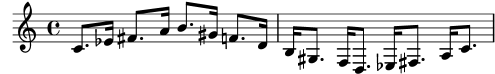### 1.1.2 Changing multiple pitches

This section discusses how to modify pitches.

#### Octave checks

In relative mode, it is easy to forget an octave changing mark. Octave checks make such errors easier to find by displaying a warning and correcting the octave if a note is found in an unexpected octave.

To check the octave of a note, specify the absolute octave after the `=` symbol. This example will generate a warning (and change the pitch) because the second note is the absolute octave `d''` instead of `d'` as indicated by the octave correction.

```\relative {
c''2 d='
e2 f
}
```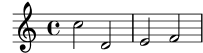The octave of notes may also be checked with the `\octaveCheck controlpitch` command. `controlpitch` is specified in absolute mode. This checks that the interval between the previous note and the `controlpitch` is within a fourth (i.e., the normal calculation of relative mode). If this check fails, a warning is printed. While the previous note itself is not changed, future notes are relative to the corrected value.

```\relative {
c''2 d
\octaveCheck c'
e2 f
}
```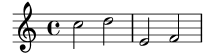Compare the two bars below. The first and third `\octaveCheck` checks fail, but the second one does not fail.

```\relative {
c''4 f g f

c4
\octaveCheck c'
f
\octaveCheck c'
g
\octaveCheck c'
f
}
```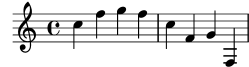Snippets: Pitches.

Internals Reference: RelativeOctaveCheck.

#### Transpose

A music expression can be transposed with `\transpose`. The syntax is

```\transpose frompitch topitch musicexpr
```

This means that `musicexpr` is transposed by the interval between the pitches `frompitch` and `topitch`: any note with pitch `frompitch` is changed to `topitch` and any other note is transposed by the same interval. Both pitches are entered in absolute mode.

Note: Music inside a `\transpose` block is absolute unless a `\relative` is included in the block.

Consider a piece written in the key of D-major. It can be transposed up to E-major; note that the key signature is automatically transposed as well.

```\transpose d e {
\relative {
\key d \major
d'4 fis a d
}
}
```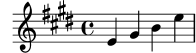If a part written in C (normal concert pitch) is to be played on the A clarinet (for which an A is notated as a C and thus sounds a minor third lower than notated), the appropriate part will be produced with:

```\transpose a c' {
\relative {
\key c \major
c'4 d e g
}
}
```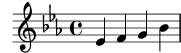Note that we specify `\key c \major` explicitly. If we do not specify a key signature, the notes will be transposed but no key signature will be printed.

`\transpose` distinguishes between enharmonic pitches: both `\transpose c cis` or `\transpose c des` will transpose up a semitone. The first version will print sharps and the notes will remain on the same scale step, the second version will print flats on the scale step above.

```music = \relative { c' d e f }
\new Staff {
\transpose c cis { \music }
\transpose c des { \music }
}
```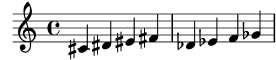`\transpose` may also be used in a different way, to input written notes for a transposing instrument. The previous examples show how to enter pitches in C (or concert pitch) and typeset them for a transposing instrument, but the opposite is also possible if you for example have a set of instrumental parts and want to print a conductor’s score. For example, when entering music for a B-flat trumpet that begins on a notated E (concert D), one would write:

```musicInBflat = { e4 … }
\transpose c bes, \musicInBflat
```

To print this music in F (e.g., rearranging to a French horn) you could wrap the existing music with another `\transpose`:

```musicInBflat = { e4 … }
\transpose f c' { \transpose c bes, \musicInBflat }
```

#### Transposing pitches with minimum accidentals ("Smart" transpose)

This example uses some Scheme code to enforce enharmonic modifications for notes in order to have the minimum number of accidentals. In this case, the following rules apply:

Double accidentals should be removed

B sharp -> C

E sharp -> F

C flat -> B

F flat -> E

In this manner, the most natural enharmonic notes are chosen.

```#(define (naturalize-pitch p)
(let ((o (ly:pitch-octave p))
(a (* 4 (ly:pitch-alteration p)))
;; alteration, a, in quarter tone steps,
;; for historical reasons
(n (ly:pitch-notename p)))
(cond
((and (> a 1) (or (eqv? n 6) (eqv? n 2)))
(set! a (- a 2))
(set! n (+ n 1)))
((and (< a -1) (or (eqv? n 0) (eqv? n 3)))
(set! a (+ a 2))
(set! n (- n 1))))
(cond
((> a 2) (set! a (- a 4)) (set! n (+ n 1)))
((< a -2) (set! a (+ a 4)) (set! n (- n 1))))
(if (< n 0) (begin (set! o (- o 1)) (set! n (+ n 7))))
(if (> n 6) (begin (set! o (+ o 1)) (set! n (- n 7))))
(ly:make-pitch o n (/ a 4))))

#(define (naturalize music)
(let ((es (ly:music-property music 'elements))
(e (ly:music-property music 'element))
(p (ly:music-property music 'pitch)))
(if (pair? es)
(ly:music-set-property!
music 'elements
(map naturalize es)))
(if (ly:music? e)
(ly:music-set-property!
music 'element
(naturalize e)))
(if (ly:pitch? p)
(begin
(set! p (naturalize-pitch p))
(ly:music-set-property! music 'pitch p)))
music))

naturalizeMusic =
#(define-music-function (m)
(ly:music?)
(naturalize m))

music = \relative c' { c4 d e g }

\score {
\new Staff {
\transpose c ais { \music }
\naturalizeMusic \transpose c ais { \music }
\transpose c deses { \music }
\naturalizeMusic \transpose c deses { \music }
}
\layout { }
}
```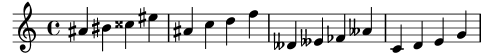Notation Reference: Instrument transpositions, Inversion, Modal transformations, Relative octave entry, Retrograde.

Snippets: Pitches.

Internals Reference: TransposedMusic.

#### Known issues and warnings

The relative conversion will not affect `\transpose`, `\chordmode` or `\relative` sections in its argument. To use relative mode within transposed music, an additional `\relative` must be placed inside `\transpose`.

Triple accidentals will not be printed if using `\transpose`. An ‘enharmonically equivalent’ pitch will be used instead (e.g., d-flat rather than e-triple-flat).

#### Inversion

A music expression can be inverted and transposed in a single operation with:

```\inversion around-pitch to-pitch musicexpr
```

The `musicexpr` is inverted interval by interval around `around-pitch`, and then transposed so that `around-pitch` is mapped to `to-pitch`.

```music = \relative { c' d e f }
\new Staff {
\music
\inversion d' d' \music
\inversion d' ees' \music
}
```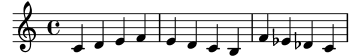Note: Motifs to be inverted should be expressed in absolute form or be first converted to absolute form by enclosing them in a `\relative` block.

Notation Reference: Modal transformations, Retrograde, Transpose.

A music expression can be reversed to produce its retrograde:

```music = \relative { c'8. ees16( fis8. a16 b8.) gis16 f8. d16 }

\new Staff {
\music
}
```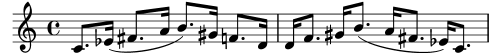#### Known issues and warnings

`\retrograde` is a rather simple tool. Since many events are ‘mirrored’ rather than exchanged, tweaks and directional modifiers for opening spanners need to be added at the matching closing spanners: `^(` needs to be ended by `^)`, every `\<` or `\cresc` needs to be ended by `\!` or `\endcr`, every `\>` or `\decr` needs to be ended by `\enddecr`. Property-changing commands/overrides with a lasting effect will likely cause surprises.

Notation Reference: Inversion, Modal transformations, Transpose.

#### Modal transformations

In a musical composition that is based on a scale, a motif is frequently transformed in various ways. It may be transposed to start at different places in the scale or it may be inverted around a pivot point in the scale. It may also be reversed to produce its retrograde, see Retrograde.

Note: Any note that does not lie within the given scale will be left untransformed.

#### Modal transposition

A motif can be transposed within a given scale with:

```\modalTranspose from-pitch to-pitch scale motif
```

The notes of motif are shifted within the scale by the number of scale degrees given by the interval between to-pitch and from-pitch:

```diatonicScale = \relative { c' d e f g a b }
motif = \relative { c'8 d e f g a b c }

\new Staff {
\motif
\modalTranspose c f \diatonicScale \motif
\modalTranspose c b, \diatonicScale \motif
}
```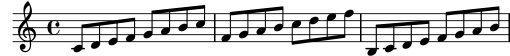An ascending scale of any length and with any intervals may be specified:

```pentatonicScale = \relative { ges aes bes des ees }
motif = \relative { ees'8 des ges,4 <ges' bes,> <ges bes,> }

\new Staff {
\motif
\modalTranspose ges ees' \pentatonicScale \motif
}
```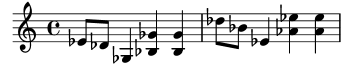When used with a chromatic scale `\modalTranspose` has a similar effect to `\transpose`, but with the ability to specify the names of the notes to be used:

```chromaticScale = \relative { c' cis d dis e f fis g gis a ais b }
motif = \relative { c'8 d e f g a b c }

\new Staff {
\motif
\transpose c f \motif
\modalTranspose c f \chromaticScale \motif
}
```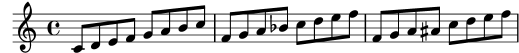#### Modal inversion

A motif can be inverted within a given scale around a given pivot note and transposed in a single operation with:

```\modalInversion around-pitch to-pitch scale motif
```

The notes of motif are placed the same number of scale degrees from the around-pitch note within the scale, but in the opposite direction, and the result is then shifted within the scale by the number of scale degrees given by the interval between to-pitch and around-pitch.

So to simply invert around a note in the scale use the same value for around-pitch and to-pitch:

```octatonicScale = \relative { ees' f fis gis a b c d }
motif = \relative { c'8. ees16 fis8. a16 b8. gis16 f8. d16 }

\new Staff {
\motif
\modalInversion fis' fis' \octatonicScale \motif
}
```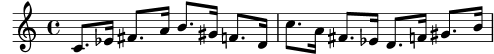To invert around a pivot between two notes in the scale, invert around one of the notes and then transpose by one scale degree. The two notes specified can be interpreted as bracketing the pivot point:

```scale = \relative { c' g' }
motive = \relative { c' c g' c, }

\new Staff {
\motive
\modalInversion c' g' \scale \motive
}
```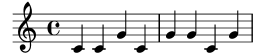```octatonicScale = \relative { ees' f fis gis a b c d }
motif = \relative { c'8. ees16 fis8. a16 b8. gis16 f8. d16 }

\new Staff {
\motif
\retrograde \modalInversion c' c' \octatonicScale \motif
}
```